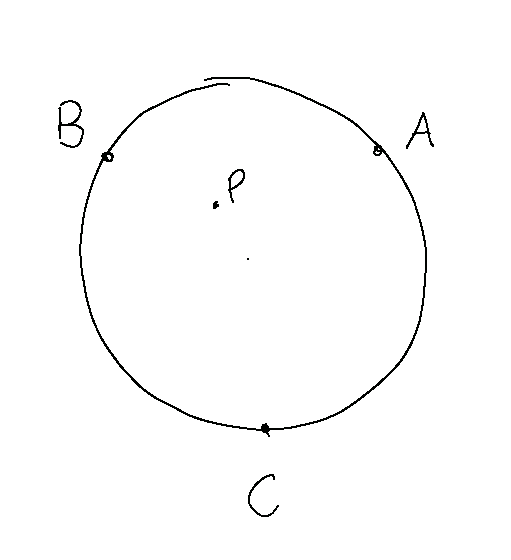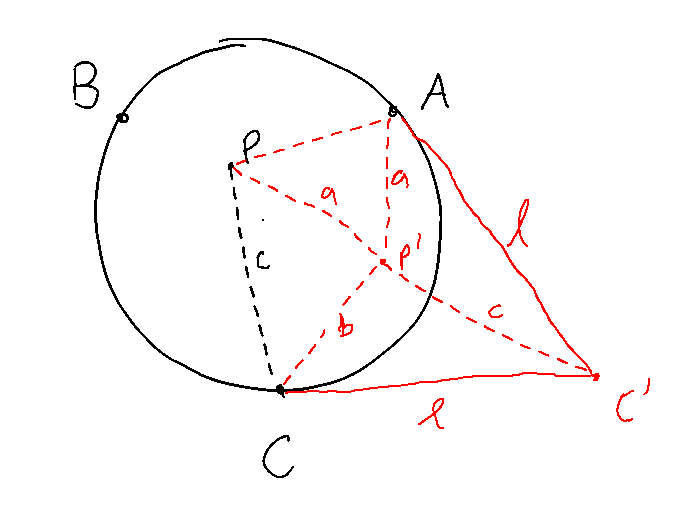Published September 7, 20202 minute read

Update 2020-09-08: This solution has been added to Kiran Kedlaya’s solution page.

I've found an elegant geometry-only proof of Putnam 2003 B5, which, to the best of my knowledge, hasn't yet been discovered.

We begin with a diagram of the problem:Rotate the diagram $60 \degree$ counterclockwise about $A$:At this point, we see that the triangle $PCP'$ has sidelengths $a$, $b$, and $c$, so these sidelengths do indeed form a triangle as claimed. Next, rotate the original diagram $60\degree$ counterclockwise about $C$:And finally, about $B$:At this point, we have a large equilateral triangle with sidelength $2\ell$, partitioned into twelve smaller triangles:

1. three triangles with sidelengths $a, b, c$,
2. two triangles with sidelengths $a, b, \ell$,
3. two triangles with sidelengths $a, c, \ell$,
4. two triangles with sidelengths $b, c, \ell$,
5. and three equilateral triangles, with sidelengths $a, b, c$ respectively.

The triangles of type 2, 3, and 4 fit together neatly to form two equilateral triangles of sidelength $\ell$. We therefore have the following decomposition:In particular, the area of the triangle we’re looking for is entirely determined by the combined area of the three equilateral triangles with sidelengths $a$, $b$, and $c$. This combined area is proportional to $a^2 + b^2 + c^2$. Treating all points as vectors in $\mathbb{R}^2$, we obtain

\begin{aligned} &a^2 + b^2 + c^2 \\ &= (A-P)^2 + (B-P)^2 + (C - P)^2 \\ &= A^2 + B^2 + C^2 + 3P^2 - 2 \, (A + B + C) \cdot P \\ &= (A^2 + B^2 + C^2 - 3 O^2) + 3 \, (P - O)^2, \end{aligned}which is entirely determined by the length $OP$, as desired.

## Footnotes

1. Strictly speaking, the decomposition works as shown if the original point lies within the triangle $ABC$. If not, then the proof still works using signed areas (but is a bit harder to follow).